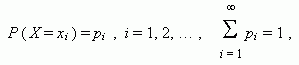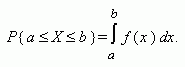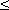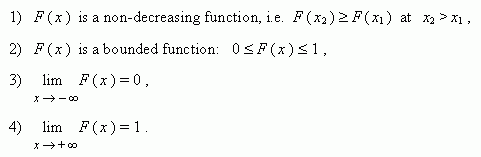Random variables

Random variable. Discrete and continuous random variables.

Independent random variables. Density function.

Distribution function. General properties of distribution function.

A variable is called random , if it can receive real values with definite probabilities as a result of experiment. The random variable X is called discrete , if such non-negative function existswhich determines the correspondence between a value õ i of the variable Õ and the probability ð i , that X receives this value.

Discrete random variables X and Y are called independent random variables , if the events Õ = õ i and Y = y j are independent for arbitrary i and j .

The random variable X is called a continuous random variable , if for any numbers a < b such non-negative function f ( x ) exists, thatThe function f ( x ) is called a density function of continuous random variable . The probability of the fact that a random variable X receives a value less than x , is called a distribution function of random variable X and marked as F ( x ) :

F ( x ) = Ð ( Xx ) .

General properties of distribution function: